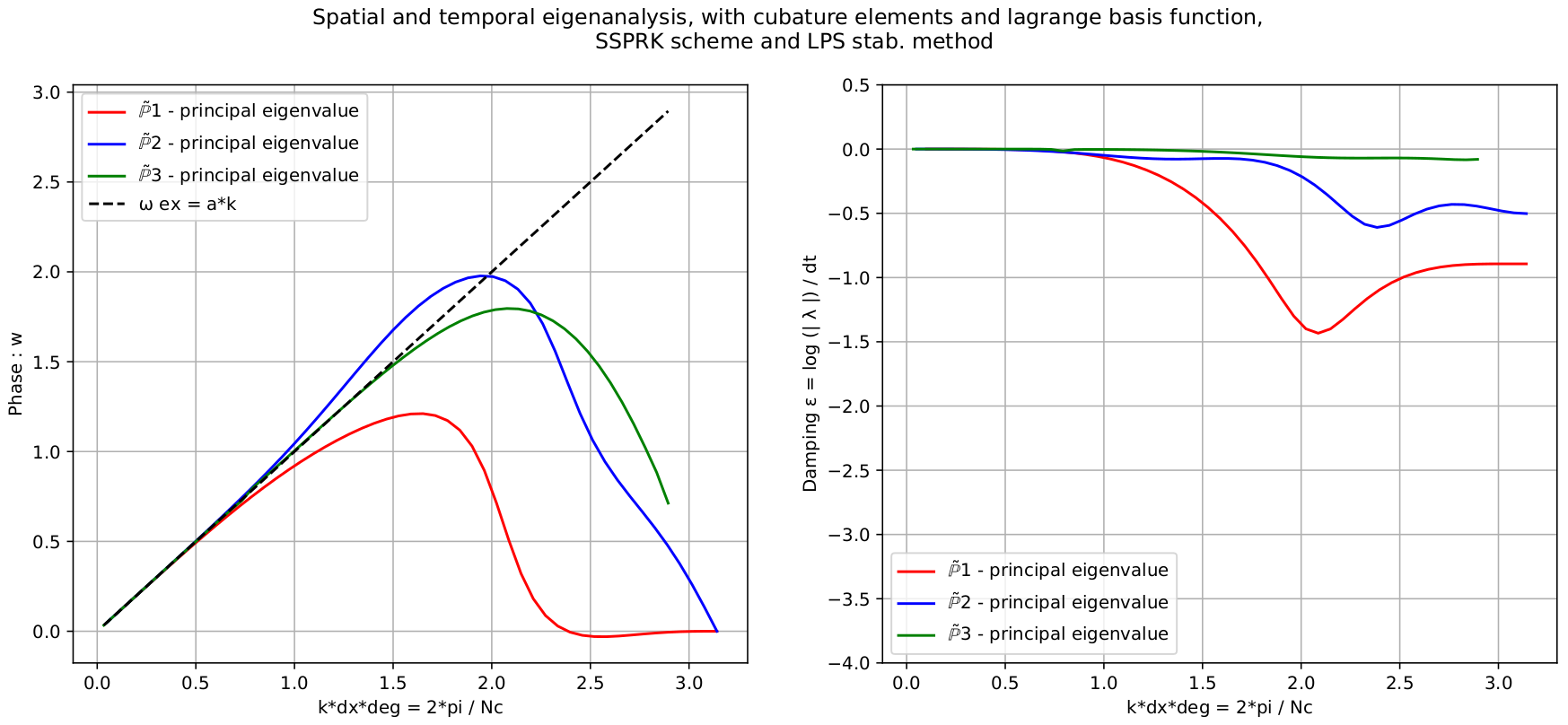# Spectral analysis of continuous FEM for hyperbolic PDEs: influence of approximation, stabilization, and time-stepping

Published in Journal of Scientific Computing, 2022

Recommended citation: Michel, S., Torlo, D., Ricchiuto, M. and Abgrall, R.. Spectral analysis of high order continuous FEM for hyperbolic PDEs on triangular meshes: influence of approximation, stabilization, and time-stepping. J Sci Comput 94, 49 (2023). https://doi.org/10.1007/s10915-022-02087-0

This is a work in collaboration with Sixtine Michel, Mario Ricchiuto and Rémi Abgrall.

In this work we study various continuous finite element discretization for two dimensional hyperbolic partial differential equations, varying the polynomial space (Lagrangian on equispaced, Lagrangian on quadrature points (Cubature) and Bernstein), the stabilization techniques (streamline-upwind Petrov-Galerkin, continuous interior penalty, orthogonal subscale stabilization) and the time discretization (Runge-Kutta (RK), strong stability preserving RK and deferred correction). This is an extension of the one dimensional study by Michel S. et al J. Sci. Comput. (2021), whose results do not hold in multi-dimensional frameworks. The study ranks these schemes based on efficiency (most of them are mass-matrix free), stability and dispersion error, providing the best CFL and stabilization coefficients. The challenges in two-dimensions are related to the Fourier analysis. Here, we perform it on two types of periodic triangular meshes varying the angle of the advection, and we combine all the results for a general stability analysis. Furthermore, we introduce additional high order viscosity to stabilize the discontinuities, in order to show how to use these methods for tests of practical interest. All the theoretical results are thoroughly validated numerically both on linear and non-linear problems, and error-CPU time curves are provided. Our final conclusions suggest that Cubature elements combined with SSPRK and OSS stabilization is the most promising combination.